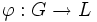# Every group admits an initial homomorphism to a pi-powered group

## Statement

Suppose$G$ is a group and$\pi$ is a set of primes. There exists a$\pi$-powered group$K$ and a homomorphism of groups$\alpha:G \to K$ such that for any$\pi$-powered group$L$ and any homomorphism$\varphi:G \to L$, there is a unique homomorphism$\theta:K \to L$ such that$\varphi = \theta \circ \alpha$.

We can think of the functor that sends$G$ to the group$K$ as the free$\pi$-powering functor. It is the left-adjoint functor to the forgetful functor from$\pi$-powered groups to groups.

### In the localization terminology

The term$\pi$-local is sometimes used to refer to a group that is powered over all the primes not in$\pi$. The functor described above is, in that context, termed the$\pi'$-localization functor. By$\pi'$ we mean the complement of$\pi$ in the set of all primes.

## Related facts

• The free powered group for a set of primes can be thought of as being obtained from the abstract free group by applying the free$\pi$-powering functor.

### Embeddability results

We are interested in cases where the canonical homomorphism from the group to its$\pi$-powering is injective, and in related questions, some of which are explored below.

Group assumption on both the starting group and the big group Divisibility/powering/torsion assumption on the starting group Divisibility/powering/torsion assumption on the big group Is this always possible? Proof
nilpotent group none divisible group No nilpotent group need not be embeddable in a divisible nilpotent group
nilpotent group$\pi$-torsion-free group (equivalently,$\pi$-powering-injective group)$\pi$-powered group Yes every pi-torsion-free nilpotent group can be embedded in a unique minimal pi-powered nilpotent group
arbitrary group none divisible group Yes every group is a subgroup of a divisible group, every pi-group is a subgroup of a divisible pi-group
arbitrary group$\pi$-torsion-free group (equivalently,$\pi$-powering-injective group)$\pi$-powered group No Powering-injective group need not be embeddable in a rationally powered group